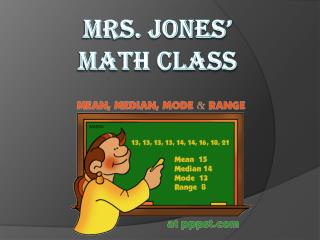Download PresentationMrs. Jones’ Math ClassMrs. Jones’ Math Class - PowerPoint PPT Presentation

Download PresentationMrs. Jones’ Math Class
An Image/Link below is provided (as is) to download presentation

Download Policy: Content on the Website is provided to you AS IS for your information and personal use and may not be sold / licensed / shared on other websites without getting consent from its author. While downloading, if for some reason you are not able to download a presentation, the publisher may have deleted the file from their server.

- - - - - - - - - - - - - - - - - - - - - - - - - - - E N D - - - - - - - - - - - - - - - - - - - - - - - - - - -
Presentation Transcript

1. Mrs. Jones’ Math Class

2. Vocabulary Words • Mean – Average of a group of numbers. • Mode – The number that appears the most times in a sequence of numbers. • Median – The number that is in the middle in a sequence of numbers in order from least to greatest. • Range - The difference between the highest and lowest numbers in the sequence.

3. Mean – The Average. Find the sum of the numbers in the sequence. Divide the sum of the numbers in the sequence by the amount of numbers that are in the sequence.

4. Mean What is the mean of the following numbers? 7, 2, 5, 6, 8, 10, 3, 4, 5, 10. The sum of the numbers is 60. There are 10 number in the sequence. The mean is 6.

5. Mode • The most “popular” number in the sequence. • There may be more than 1 mode!!!!

6. Mode • What is the mode in this number sequence?3, 4, 5, 3, 2, 2, 5, 5, 6, 10, 5 The mode in this number sequence is 5.

7. Median • The middle number in a number sequence that is in order from least to greatest.

8. Median • Find the median. • Remember, the numbers have to be in order from lowest to greatest. 2, 3, 1, 6, 5, 8, 7. - Put these in order first. 1, 2, 3, 5, 6, 7, 8 • The median is 5.

9. Range • The difference of the highest and lowest numbers in the sequence.

10. Range • What is the range for the following number sequence?3, 5, 6, 8, 10, 35, 48, 104104 – 3 = 101 • The range is 101.

11. References • http://blogs.parisisd.net/crawdadteacher1/2007/07/18/math-powerpoints • http://jc-schools.net/ce/ppt6-12math.htm • http://www.quia.com/pages/hostettermathcs.html#add • www.easycalculation.com • www.ppst.com

12. quiz • What is range? • How do you find median? • What is the difference between median and mean? • How do you find mode in a sequence of numbers? • What is the range in this sequence? - 2, 3, 5, 7, 8, 10.